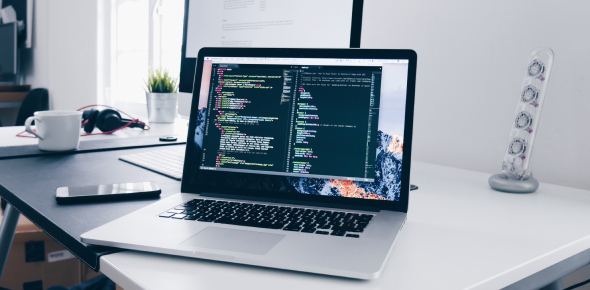# Computer Fundamentals Semester 2 Test 2

20 Questions | Total Attempts: 38Settings• 1.
The four stages of the design process are:
1. Investigate the problem
2. Design the solution
3. Produce the solution
4. Evaluate the solution
Imagine you are a website developer and your next job is to set-up a website for Jim who runs a lawnmowing and gardening business.  What would you do at each stage of the design process in this job? You should write at least one full sentence for each of the four points above.
• 2.
Hyperlinking is creating a link from one webpage or document to another. Explain how to insert a hyperlink (for example, in our Ultranet Collaborative Space).
• 3.
What sort of information can be entered in a database? (You can select more than one)
• A.

Videos

• B.

Text

• C.

Images

• D.

Numbers

• 4.
Applying a FILTER in a database table reduces the number of records visible.
• A.

True

• B.

False

• 5.
How can computers and devices be connected in a network?
• A.

By a modem

• B.

By a network cable

• C.

By wireless

• D.

All of these

• E.

None of these

• 6.
Which of these IS NOT an advantage of a computer network
• A.

Saving electricity

• B.

Sharing resources

• C.

Sharing programs

• D.

Communicating

• 7.
One advantage of a BUS network is that is uses less cabling
• A.

True

• B.

False

• 8.
This is a drawing of a
• A.

Bus network

• B.

Star network

• C.

Peer-to-peer network

• D.

None of these

• 9.
Which type of network is unaffected if one of the computers on it fails?
• A.

Bus network

• B.

Star network

• 10.
If you find an image online that has a Creative Commons Attribution licence what can you do with it?
• A.

Nothing

• B.

Only use it in a print document

• C.

Post it online

• D.

Use it online or in print with attribution to the photographer

• 11.
What is a single sheet in an Excel file called?
• A.

Worksheet

• B.

Workbook

• C.

• D.

Column

• 12.
Software and storage located on the Internet and accessed via a web browser is called  _____ computing?
• 13.
In Visual Basic Express the appearance of a button, label or textbox can be changed in the
• A.

Controls

• B.

Properties

• C.

Form

• D.

Code

• 14.
In Visual Basic Express the function of a button or other control is edited in
• A.

Properties

• B.

Control

• C.

Code

• D.

Form

• 15.
In Excel what is the correct formula to calculate the total of cells B3 and B4?
• A.

Summary(b3+b4)

• B.

SUM B4+B3

• C.

SUMMARY(B3+B4)

• D.

SUM(B3+B4)

• E.

TOTAL(B3+B4)

• 16.
Which of these is NOT a type of image file?
• A.

JPG

• B.

PNG

• C.

FLN

• D.

JPEG

• 17.
Which of these can be made/done using Excel? (You can select more than one)
• A.

Charts

• B.

Calculations

• C.

Drawings

• D.

Animations

• E.

Organise information

• 18.
What is the address of this cell?
Related TopicsBack to top